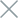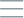• Home
• Tags
• Series
•# Heuristic Search Algorithm in C++ - Explanation, Example and Code

Heuristic Search is a powerful algorithmic approach used to find solutions in complex problem spaces by making informed decisions based on heuristics or rules of thumb. It's particularly useful when an exhaustive search is impractical due to the large search space.

## How It Works

1. Heuristic Evaluation: The algorithm evaluates each state in the problem space using a heuristic function. This function estimates the "promisingness" of each state in terms of its closeness to the goal state.
2. Search Strategy: The algorithm selects the most promising state based on the heuristic evaluation. It uses a search strategy like Best-First Search, A* Search, or Greedy Search.
3. State Expansion: The selected state is expanded by generating its neighboring states. These are potential candidates for the next step.
4. Repeat: The process is repeated iteratively, selecting and expanding states until the goal state is found or a termination condition is met.

## Example: Traveling Salesman Problem (TSP)

Consider the Traveling Salesman Problem, where a salesman needs to visit a set of cities and return to the starting city while minimizing the total distance traveled. A heuristic approach could be the Nearest Neighbor Algorithm:

1. Start at a random city.
2. At each step, choose the nearest unvisited city as the next destination.
3. Repeat until all cities are visited, then return to the starting city.

## Code Example in C++

``````#include <iostream>
#include <vector>
#include <cmath>
#include <algorithm>

struct City {
int x, y;
};

double distance(const City& city1, const City& city2) {
return std::sqrt(std::pow(city1.x - city2.x, 2) + std::pow(city1.y - city2.y, 2));
}

std::vector<int> nearestNeighbor(const std::vector<City>& cities) {
int numCities = cities.size();
std::vector<int> path(numCities);
std::vector<bool> visited(numCities, false);

path = 0;
visited = true;

for (int i = 1; i < numCities; ++i) {
int currentCity = path[i - 1];
double minDist = std::numeric_limits<double>::max();
int nextCity = -1;

for (int j = 0; j < numCities; ++j) {
if (!visited[j]) {
double dist = distance(cities[currentCity], cities[j]);
if (dist < minDist) {
minDist = dist;
nextCity = j;
}
}
}

path[i] = nextCity;
visited[nextCity] = true;
}

return path;
}

int main() {
std::vector<City> cities = {{0, 0}, {1, 3}, {4, 2}, {3, 6}, {7, 1}};
std::vector<int> path = nearestNeighbor(cities);

std::cout << "Traveling Salesman Path: ";
for (int city : path) {
std::cout << city << " ";
}
std::cout << std::endl;

return 0;
}
``````

In this example, the Nearest Neighbor Algorithm is used to solve the Traveling Salesman Problem. It's a heuristic approach that chooses the nearest unvisited city at each step, resulting in a solution that's often close to optimal.